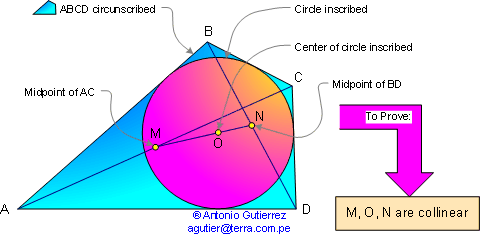Newton's Theorem: Newton's line

In a circumscribed quadrilateral the center of the circle inscribed lies on the line joining the midpoints of the diagonals, called Newton's line.## Hints

1. In any quadrilateral ABCD that is not a parallelogram , if O' lies on MN prove that:
Area(AO'B) + Area(CO'D) = Area(AO'D) + Area(BO'C)

2. In a circumscribed quadrilateral ABCD if O is the center of the circle inscribed prove that:
Area(AOB) + Area(COD) = Area(AOD) + Area(BOC)

3. From (1) and (2), O lies on MN.

See also: Puzzle of the Newton's Theorem: 50 pieces of circles.

 Home  | Collinear Points | Email Last updated: July 8, 2009